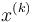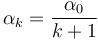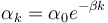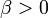# Gradient descent with decaying learning rate

(diff) ← Older revision | Latest revision (diff) | Newer revision → (diff)

## Definition

Gradient descent with decaying learning rate is a form of gradient descent where the learning rate varies as a function of the number of iterations, but is not otherwise dependent on the value of the vector at the stage. The update rule is as follows:

$x}^{(k+1)} = \vec{x}^{(k)} - \alpha_k f\left(\vec{x^{(k)$

where$\alpha_k$ depends only on$k$ and not on the choice of$x^{(k)}$.

## Cases

Type of decay Example expression for$\alpha_k$ More information
linear decay$\alpha_k = \frac{\alpha_0}{k + 1}$ Gradient descent with linearly decaying learning rate
quadratic decay$\alpha_k = \frac{\alpha_0}{(k + 1)^2}$ Gradient descent with quadratically decaying learning rate
exponential decay$\alpha_k = \alpha_0 e^{-\beta k}$ where$\beta > 0$ Gradient descent with exponentially decaying learning rate